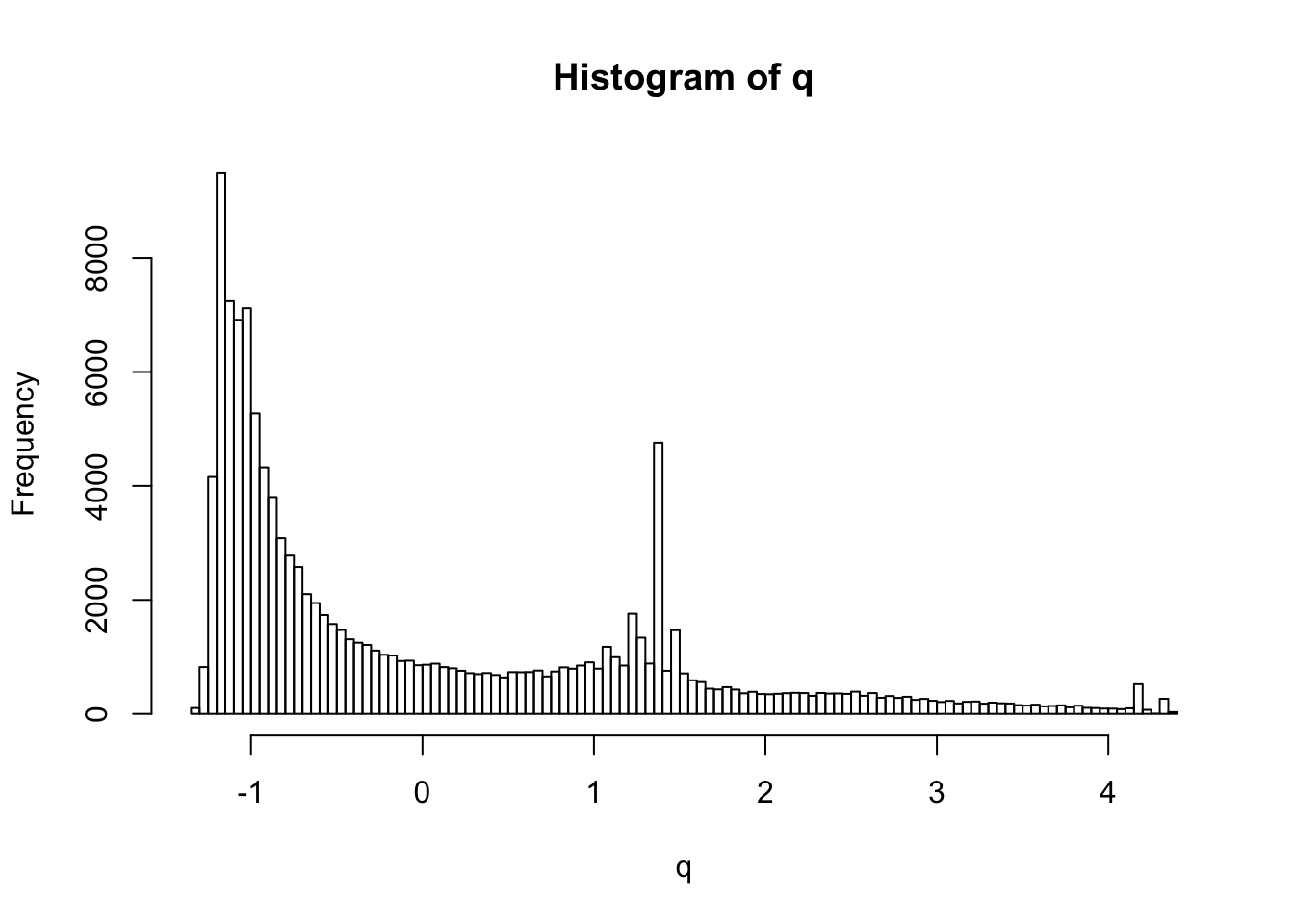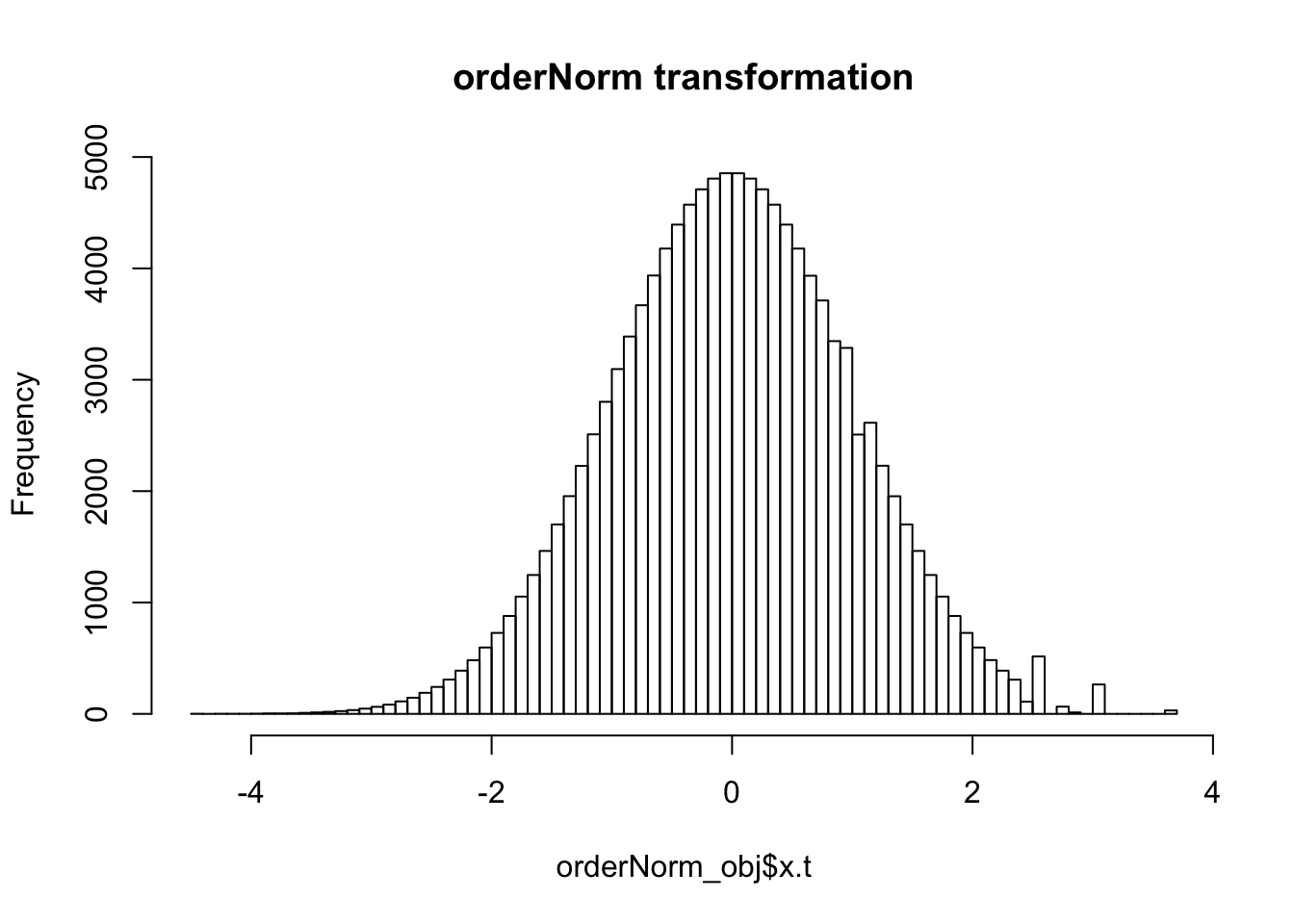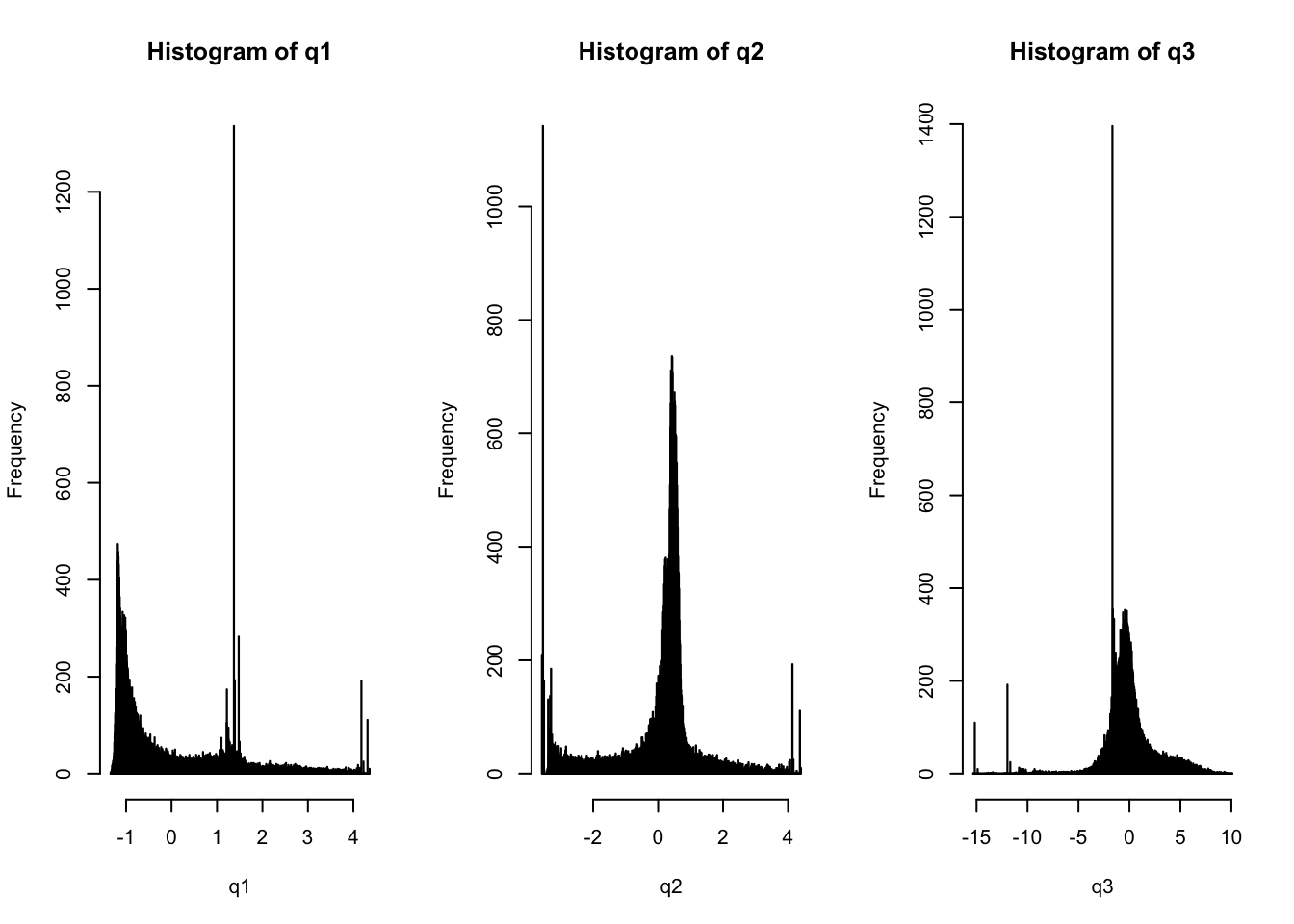## 1. cFDR

### Transform q values to normal distribution

A quick fix would be to convert the q values to a normal distribution and then directly use James’ functions.

The `bestNormalize` package (https://cran.r-project.org/web/packages/bestNormalize/vignettes/bestNormalize.html) allows comparison between different normalisation methods (where normalisation refers to making data normal, i.e. not just \([0,1]\)).

``````mca_df <- readRDS("res.mca.mjca.RDS")
q <- mca_df[,1]
hist(q, breaks = 100)``````I use the package to find the recommended method for normalisation. The package suggests the ordered quantile (ORQ) normalization via `orderNorm`, a normalization technique based off of a rank mapping to the normal distribution, which guarantees normally distributed transformed data (if ties are not present).

``````library(bestNormalize)
(BNobject <- bestNormalize(q))``````
``## Warning in bestNormalize(q): boxcox  did not work;  Error in estimate_boxcox_lambda(x, ...) : x must be positive``
``## Warning in orderNorm(standardize = TRUE, warn = TRUE, x = c(1.23253769877005, : Ties in data, Normal distribution not guaranteed``
``````## Best Normalizing transformation with 121879 Observations
##  Estimated Normality Statistics (Pearson P / df, lower => more normal):
##  - No transform: 248.1234
##  - Log_b(x+a): 62.6722
##  - sqrt(x+a): 91.8964
##  - exp(x): 2154.9042
##  - arcsinh(x): 292.3957
##  - Yeo-Johnson: 132.1966
##  - orderNorm: 4.7114
## Estimation method: Out-of-sample via CV with 10 folds and 5 repeats
##
## Based off these, bestNormalize chose:
## orderNorm Transformation with 121879 nonmissing obs and ties
##  - 102874 unique values
##  - Original quantiles:
##     0%    25%    50%    75%   100%
## -1.344 -1.038 -0.620  0.997  4.357``````

The annotation data is now normally distributed.

``orderNorm_obj <- orderNorm(q)``
``## Warning in orderNorm(q): Ties in data, Normal distribution not guaranteed``
``hist(orderNorm_obj\$x.t, main = "orderNorm transformation", breaks = 100)``### Problem

James’ code does not allow p or q \(<1e-300\) which corresponds to 50% of the dataset.

``````final_dataset <- readRDS("final_dataset.RDS")

p <- final_dataset\$p

source("cfdr_functions.R")

# p[which(p<1e-300)] <- 1e-300 # to avoid errors in James' code
# q[which(qs<1e-300)] <- 1e-300

candidate_indices <- which(p < 1)

chr <- final_dataset\$chrom

est_q0_pars <- fit.2g(q[which(p>0.5)])\$pars

# prepare containers
fold <- vector(mode = "list", length = length(unique(chr)))
ind <- vector(mode = "list", length = length(unique(chr)))
L <- vector(mode = "list", length = length(unique(chr)))
v <- vector(mode = "list", length = length(unique(chr)))

for(i in 1:length(unique(chr))){
fold[[i]] <- which(chr == i)
ind[[i]] <- intersect(candidate_indices, fold[[i]])
L[[i]] <- vl(p, q, indices=ind[[i]], mode=2, fold=fold[[i]], gx = min(p[ind[[i]]]));  # compute L curves

v[[i]] <- il(L[[i]], pi0_null = est_q0_pars, sigma_null = est_q0_pars, distx="norm", gx = min(p[ind[[i]]])) # integrate over L curves
}

save(p, q, v, file = "transform_res.RData")``````

### New method

We need to:

• Fit a density estimate to the Q data.
• Use that same density estimate in generating the L curves.

#### Density estimation

We need to approximate the density of \(f_0(p,q)=f(P=p, Q=q|H_0^p)=f(Q=q|H_0^p)\). To do this we consider the distribution of Q where \(P>1/2\).

``````final_dataset <- readRDS("final_dataset.RDS")
p <- final_dataset\$p
ind <- which(p>1/2)

q1 <- mca_df[,1][ind]
q2 <- mca_df[,2][ind]
q3 <- mca_df[,3][ind]

par(mfrow=c(1,3))
hist(q1, breaks = 1000)
hist(q2, breaks = 1000)
hist(q3, breaks = 1000)``````Notice that there are spikes at the edges of the distribution (for q2 and q3) which may cause us problems. We need a density estimator that extends beyond the data boundaries if needed (e.g. dimensions 2 and 3).

Idea: Try Gaussian mixture models for density estimation. To do this I’ll use the `mclust` R package.

#### Gaussian mixture models

• Assume that all our data points come from one of \(k\) Gaussian distributions (with different means and variances).

• Note that this is different to the \(K\)-means algorithm, where the clusters are modelled only by their mean.

• EM algorithm assigns data to clusters with some probability and is used to infer the parameter values.

• E-step (“Expectation”): Treats means, covariances and weights as fixed. For each data point, calculate the probability that it belongs to each Gaussian and treat these as the relative “assignment probabilities” for each of the Gaussians for that data point.

• M-step (“Maximum”): Treat the assignment probabilities as fixed and update the parameters (mean, covariancee and weights) using these. E.g. the mean of cluster C is the mean of the data points weighted by their assignment probabilities to cluster C.

• Repeat E-M steps until convergence.

#### Q1 (Dimension 1)

``````par(mfrow=c(2,2))
q1_mclust <- densityMclust(q1)
summary(q1_mclust)``````
``````## -------------------------------------------------------
## Density estimation via Gaussian finite mixture modeling
## -------------------------------------------------------
##
## Mclust V (univariate, unequal variance) model with 7 components:
##
##  log-likelihood     n df       BIC       ICL
##       -46180.02 49359 20 -92576.18 -116196.8``````
``````plot(q1_mclust, what = "BIC") # E is equal variance, V is variable variance
plot(q1_mclust, what = "density", data = q1, breaks = 100)
plot(q1_mclust, what = "diagnostic", type = "cdf")
plot(q1_mclust, what = "diagnostic", type = "qq")``````
``````## Warning in regularize.values(x, y, ties, missing(ties)): collapsing to
## unique 'x' values``````